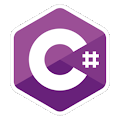Programming Course in C# ¡Free!

# Calculator - if

Proposed exercise

Write a program which asks the user for two numbers and an operation to perform on them (+,-,*,x,/) and displays the result of that operation, as in this example:

Enter first number: 5
Enter operation: +
Enter second number: 7
5+7=12

Note: you MUST use "if", not "switch"

Output

Solution

```using System;
public class CalculatorIf
{
public static void Main()
{
int a, b;
char operation;

Console.Write("Enter first number: ");
Console.Write("Enter operation: ");
Console.Write("Enter second number: ");

if (operation=='+')
Console.WriteLine("{0} + {1} = {2}", a, b, a+b);
else if (operation=='-')
Console.WriteLine("{0} - {1} = {2}", a, b, a-b);
else if ((operation=='x') || (operation=='*'))
Console.WriteLine("{0} * {1} = {2}", a, b, a*b);
else if (operation=='/')
Console.WriteLine("{0} / {1} = {2}", a, b, a/b);
else
Console.WriteLine("Wrong Character");
}
}
```